## Kinetic Theory: Atomic and Molecular Explanation of Pressure and Temperature

### Learning Objectives

By the end of this section, you will be able to:

• Express the ideal gas law in terms of molecular mass and velocity.
• Define thermal energy.
• Calculate the kinetic energy of a gas molecule, given its temperature.
• Describe the relationship between the temperature of a gas and the kinetic energy of atoms and molecules.
• Describe the distribution of speeds of molecules in a gas.

We have developed macroscopic definitions of pressure and temperature. Pressure is the force divided by the area on which the force is exerted, and temperature is measured with a thermometer. We gain a better understanding of pressure and temperature from the kinetic theory of gases, which assumes that atoms and molecules are in continuous random motion.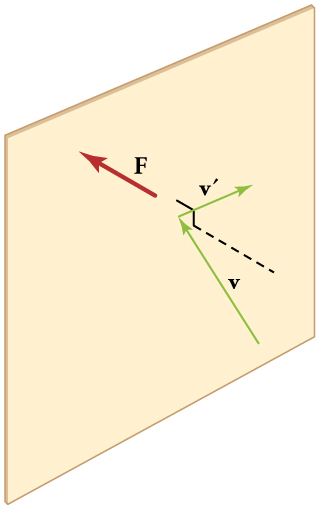Figure 1. When a molecule collides with a rigid wall, the component of its momentum perpendicular to the wall is reversed. A force is thus exerted on the wall, creating pressure.

Figure 1 shows an elastic collision of a gas molecule with the wall of a container, so that it exerts a force on the wall (by Newton’s third law). Because a huge number of molecules will collide with the wall in a short time, we observe an average force per unit area. These collisions are the source of pressure in a gas. As the number of molecules increases, the number of collisions and thus the pressure increase. Similarly, the gas pressure is higher if the average velocity of molecules is higher. The actual relationship is derived in the Making Connections feature below. The following relationship is found: $PV=\frac{1}{3}Nm{\overline{v^2}}\\$, where P is the pressure (average force per unit area), V is the volume of gas in the container, N is the number of molecules in the container, m is the mass of a molecule, and $\overline{v^2}\\$ is the average of the molecular speed squared.

What can we learn from this atomic and molecular version of the ideal gas law? We can derive a relationship between temperature and the average translational kinetic energy of molecules in a gas. Recall the previous expression of the ideal gas law: PVNkT.

Equating the right-hand side of this equation with the right-hand side of $PV=\frac{1}{3}Nm{\overline{v^2}}\\$ gives $\frac{1}{3}Nm{\overline{v^2}}=NkT\\$.

### Making Connections: Things Great and Small—Atomic and Molecular Origin of Pressure in a Gas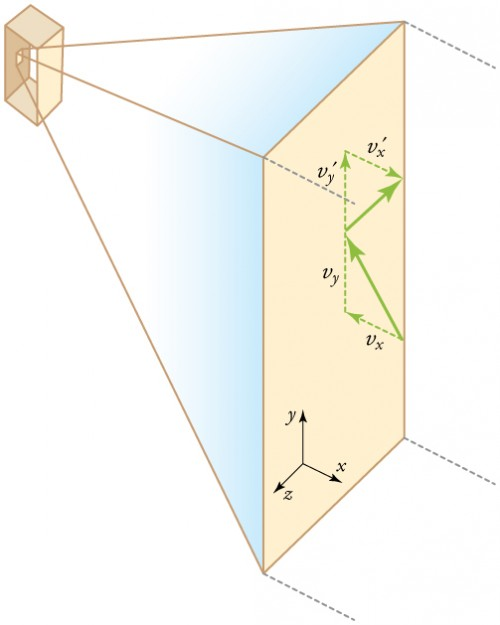Figure 2. Gas in a box exerts an outward pressure on its walls. A molecule colliding with a rigid wall has the direction of its velocity and momentum in the x-direction reversed. This direction is perpendicular to the wall. The components of its velocity momentum in the y- and z-directions are not changed, which means there is no force parallel to the wall.

Figure 2 shows a box filled with a gas. We know from our previous discussions that putting more gas into the box produces greater pressure, and that increasing the temperature of the gas also produces a greater pressure. But why should increasing the temperature of the gas increase the pressure in the box? A look at the atomic and molecular scale gives us some answers, and an alternative expression for the ideal gas law.

The figure shows an expanded view of an elastic collision of a gas molecule with the wall of a container. Calculating the average force exerted by such molecules will lead us to the ideal gas law, and to the connection between temperature and molecular kinetic energy. We assume that a molecule is small compared with the separation of molecules in the gas, and that its interaction with other molecules can be ignored. We also assume the wall is rigid and that the molecule’s direction changes, but that its speed remains constant (and hence its kinetic energy and the magnitude of its momentum remain constant as well). This assumption is not always valid, but the same result is obtained with a more detailed description of the molecule’s exchange of energy and momentum with the wall.

If the molecule’s velocity changes in the x-direction, its momentum changes from −mvx to +mvx. Thus, its change in momentum is Δmv = + mvx −(−mvx) = 2mvx. The force exerted on the molecule is given by $F=\frac{\Delta{p}}{\Delta{t}}=\frac{2mv_x}{\Delta{t}}\\$.

There is no force between the wall and the molecule until the molecule hits the wall. During the short time of the collision, the force between the molecule and wall is relatively large. We are looking for an average force; we take Δt to be the average time between collisions of the molecule with this wall. It is the time it would take the molecule to go across the box and back (a distance 2l) at a speed of vx. Thus $\Delta{t}=\frac{2l}{v_x}\\$, and the expression for the force becomes

$\displaystyle{F}=\frac{2mv_x}{\frac{2l}{v_x}}=\frac{{mv_x}^2}{l}\\$

This force is due to one molecule. We multiply by the number of molecules N and use their average squared velocity to find the force

$\displaystyle{F}=N\frac{m\overline{{v_x}^2}}{l}\\$,

where the bar over a quantity means its average value. We would like to have the force in terms of the speed v, rather than the x-component of the velocity. We note that the total velocity squared is the sum of the squares of its components, so that

$\overline{v^2}=\overline{{v_x}^2}+\overline{{v_y}^2}+\overline{{v_z}^2}\\$.

Because the velocities are random, their average components in all directions are the same:

$\overline{{v_x}^2}=\overline{{v_y}^2}=\overline{{v_z}^2}\\$.

Thus,

$\overline{v^2}=3\overline{{v_x}^2}\\$ or $\overline{{v_x}^2}=\frac{1}{3}\overline{v^2}\\$.

Substituting $\frac{1}{3}\overline{v^2}\\$ into the expression for F gives

$F=N\frac{m\overline{v^2}}{3l}\\$.

The pressure is $\frac{F}{A}\\$, so that we obtain $P=\frac{F}{A}=N\frac{m\overline{v^2}}{3Al}=\frac{1}{3}\frac{Nm\overline{v^2}}{V}\\$, where we used VAl for the volume. This gives the important result.

$PV=\frac{1}{3}Nm\overline{v^2}\\$

This equation is another expression of the ideal gas law.

We can get the average kinetic energy of a molecule, $\frac{1}{2}mv^2\\$, from the left-hand side of the equation by canceling N and multiplying by 3/2. This calculation produces the result that the average kinetic energy of a molecule is directly related to absolute temperature.

$\overline{\text{KE}}=\frac{1}{2}m\overline{v^2}=\frac{3}{2}kT\\$

The average translational kinetic energy of a molecule, $\overline{\text{KE}}\\$, is called thermal energy. The equation $\overline{\text{KE}}=\frac{1}{2}m\overline{v^2}=\frac{3}{2}kT\\$ is a molecular interpretation of temperature, and it has been found to be valid for gases and reasonably accurate in liquids and solids. It is another definition of temperature based on an expression of the molecular energy.

It is sometimes useful to rearrange $\overline{\text{KE}}=\frac{1}{2}m\overline{v^2}=\frac{3}{2}kT\\$, and solve for the average speed of molecules in a gas in terms of temperature,

$\displaystyle\sqrt{\overline{v^2}}=v_{\text{rms}}=\sqrt{\frac{3kT}{m}}\\$

where vrms stands for root-mean-square (rms) speed.

### Example 1. Calculating Kinetic Energy and Speed of a Gas Molecule

1. What is the average kinetic energy of a gas molecule at 20.0ºC (room temperature)?
2. Find the rms speed of a nitrogen molecule (N2) at this temperature.

#### Strategy for Part 1

The known in the equation for the average kinetic energy is the temperature.

$\overline{\text{KE}}=\frac{1}{2}m\overline{v^2}=\frac{3}{2}kT\\$

Before substituting values into this equation, we must convert the given temperature to kelvins. This conversion gives T = (20.0 + 273)K = 293K.

#### Solution for Part 1

The temperature alone is sufficient to find the average translational kinetic energy. Substituting the temperature into the translational kinetic energy equation gives

$\overline{\text{KE}}=\frac{3}{2}kT=\frac{3}{2}\left(1.38\times10^{-23}\text{ J/K}\right)\left(293\text{ K}\right)=6.07\times10^{-21}\text{ J}\\$

#### Strategy for Part 2

Finding the rms speed of a nitrogen molecule involves a straightforward calculation using the equation

$\displaystyle\sqrt{\overline{v^2}}=v_{\text{rms}}=\sqrt{\frac{3kT}{m}}\\$

but we must first find the mass of a nitrogen molecule. Using the molecular mass of nitrogen N2 from the periodic table,

$m=\frac{2(14.0067)\times10^{-3}\text{ kg/mol}}{6.02\times10^{23}\text{ mol}^{-1}}=4.65\times10^{-26}\text{ kg}\\$

#### Solution for Part 2

Substituting this mass and the value for k into the equation for vrms yields

$\displaystyle{v}_{\text{rms}}=\sqrt{\frac{3kT}{m}}=\sqrt{\frac{3\left(1.38\times10^{-23}\text{ J/K}\right)\left(293\text{ K}\right)}{4.65\times10^{-26}\text{ kg}}}=511\text{ m/s}\\$

#### Discussion

Note that the average kinetic energy of the molecule is independent of the type of molecule. The average translational kinetic energy depends only on absolute temperature. The kinetic energy is very small compared to macroscopic energies, so that we do not feel when an air molecule is hitting our skin. The rms velocity of the nitrogen molecule is surprisingly large. These large molecular velocities do not yield macroscopic movement of air, since the molecules move in all directions with equal likelihood. The mean free path (the distance a molecule can move on average between collisions) of molecules in air is very small, and so the molecules move rapidly but do not get very far in a second. The high value for rms speed is reflected in the speed of sound, however, which is about 340 m/s at room temperature. The faster the rms speed of air molecules, the faster that sound vibrations can be transferred through the air. The speed of sound increases with temperature and is greater in gases with small molecular masses, such as helium. (See Figure 3.)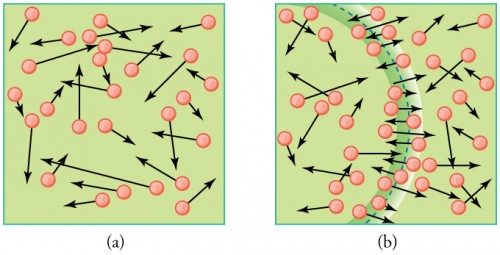Figure 3. (a) There are many molecules moving so fast in an ordinary gas that they collide a billion times every second. (b) Individual molecules do not move very far in a small amount of time, but disturbances like sound waves are transmitted at speeds related to the molecular speeds.

### Making Connections: Historical Note—Kinetic Theory of Gases

The kinetic theory of gases was developed by Daniel Bernoulli (1700–1782), who is best known in physics for his work on fluid flow (hydrodynamics). Bernoulli’s work predates the atomistic view of matter established by Dalton.

## Distribution of Molecular Speeds

The motion of molecules in a gas is random in magnitude and direction for individual molecules, but a gas of many molecules has a predictable distribution of molecular speeds. This distribution is called the Maxwell-Boltzmann distribution, after its originators, who calculated it based on kinetic theory, and has since been confirmed experimentally. (See Figure 4.) The distribution has a long tail, because a few molecules may go several times the rms speed. The most probable speed vp is less than the rms speed vrms. Figure 5 shows that the curve is shifted to higher speeds at higher temperatures, with a broader range of speeds.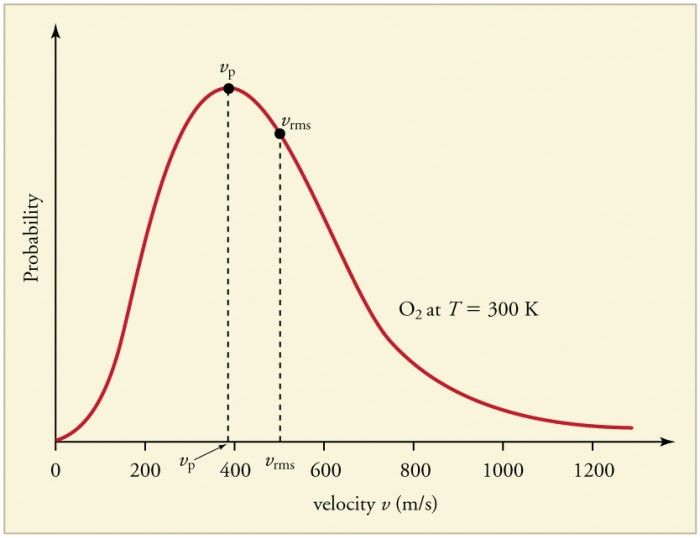Figure 4. The Maxwell-Boltzmann distribution of molecular speeds in an ideal gas. The most likely speed vp is less than the rms speed vrms. Although very high speeds are possible, only a tiny fraction of the molecules have speeds that are an order of magnitude greater than vrms.

The distribution of thermal speeds depends strongly on temperature. As temperature increases, the speeds are shifted to higher values and the distribution is broadened.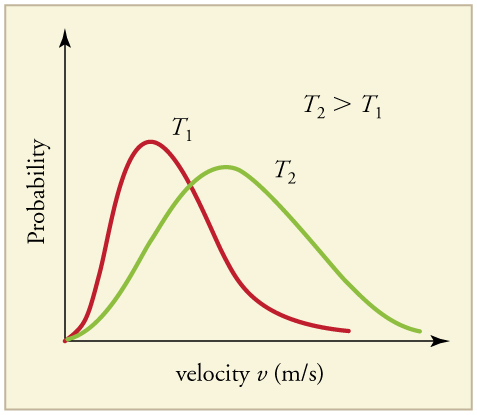Figure 5. The Maxwell-Boltzmann distribution is shifted to higher speeds and is broadened at higher temperatures.

What is the implication of the change in distribution with temperature shown in Figure 5 for humans? All other things being equal, if a person has a fever, he or she is likely to lose more water molecules, particularly from linings along moist cavities such as the lungs and mouth, creating a dry sensation in the mouth.

### Example 2. Calculating Temperature: Escape Velocity of Helium Atoms

In order to escape Earth’s gravity, an object near the top of the atmosphere (at an altitude of 100 km) must travel away from Earth at 11.1 km/s. This speed is called the escape velocity. At what temperature would helium atoms have an rms speed equal to the escape velocity?

#### Strategy

Identify the knowns and unknowns and determine which equations to use to solve the problem.

#### Solution

Identify the knowns: v is the escape velocity, 11.1 km/s.

Identify the unknowns: We need to solve for temperature, T. We also need to solve for the mass m of the helium atom.

Determine which equations are needed. To solve for mass m of the helium atom, we can use information from the periodic table:

$m=\frac{\text{molar mass}}{\text{number of atoms per mole}}\\$

To solve for temperature T, we can rearrange either $\overline{KE}=\frac{1}{2}m\overline{v^2}=\frac{3}{2}kT\\$ or $\sqrt{\overline{v^2}}=v_{\text{rms}}=\sqrt{\frac{3kT}{m}}\\$ to yield $T=\frac{m\overline{v^2}}{3k}\\$, where k is the Boltzmann constant and m is the mass of a helium atom.

Plug the known values into the equations and solve for the unknowns.

$m=\frac{\text{molar mass}}{\text{number of atoms per mole}}=\frac{4.0026\times10^{-3}\text{ kg/mol}}{6.02\times10^{23}\text{ mol}}=6.65\times10^{-27}\text{ kg}\\$

$T=\frac{\left(6.65\times10^{-27}\text{ kg}\right)\left(11.1\times10^3\text{ m/s}\right)^2}{3\left(1.38\times10^{-23}\text{ J/K}\right)}=1.98\times10^4\text{ K}\\$

#### Discussion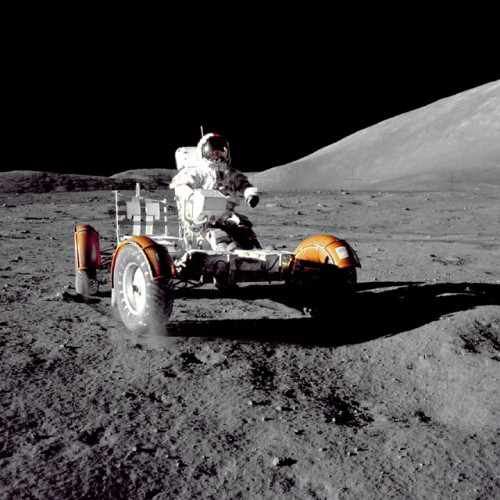Figure 6. This photograph of Apollo 17 Commander Eugene Cernan driving the lunar rover on the Moon in 1972 looks as though it was taken at night with a large spotlight. In fact, the light is coming from the Sun. Because the acceleration due to gravity on the Moon is so low (about 1/6 that of Earth), the Moon’s escape velocity is much smaller. As a result, gas molecules escape very easily from the Moon, leaving it with virtually no atmosphere. Even during the daytime, the sky is black because there is no gas to scatter sunlight. (credit: Harrison H. Schmitt/NASA)

This temperature is much higher than atmospheric temperature, which is approximately 250 K (25ºC or 10ºF) at high altitude. Very few helium atoms are left in the atmosphere, but there were many when the atmosphere was formed. The reason for the loss of helium atoms is that there are a small number of helium atoms with speeds higher than Earth’s escape velocity even at normal temperatures. The speed of a helium atom changes from one instant to the next, so that at any instant, there is a small, but nonzero chance that the speed is greater than the escape speed and the molecule escapes from Earth’s gravitational pull. Heavier molecules, such as oxygen, nitrogen, and water (very little of which reach a very high altitude), have smaller rms speeds, and so it is much less likely that any of them will have speeds greater than the escape velocity.

In fact, so few have speeds above the escape velocity that billions of years are required to lose significant amounts of the atmosphere. Figure 6 shows the impact of a lack of an atmosphere on the Moon. Because the gravitational pull of the Moon is much weaker, it has lost almost its entire atmosphere. The comparison between Earth and the Moon is discussed in this chapter’s Problems and Exercises.

### Check Your Understanding

If you consider a very small object such as a grain of pollen, in a gas, then the number of atoms and molecules striking its surface would also be relatively small. Would the grain of pollen experience any fluctuations in pressure due to statistical fluctuations in the number of gas atoms and molecules striking it in a given amount of time?

#### Solution

Yes. Such fluctuations actually occur for a body of any size in a gas, but since the numbers of atoms and molecules are immense for macroscopic bodies, the fluctuations are a tiny percentage of the number of collisions, and the averages spoken of in this section vary imperceptibly. Roughly speaking the fluctuations are proportional to the inverse square root of the number of collisions, so for small bodies they can become significant. This was actually observed in the 19th century for pollen grains in water, and is known as the Brownian effect.

## PhET Explorations: Gas Properties

Pump gas molecules into a box and see what happens as you change the volume, add or remove heat, change gravity, and more. Measure the temperature and pressure, and discover how the properties of the gas vary in relation to each other.

## Section Summary

• Kinetic theory is the atomistic description of gases as well as liquids and solids.
• Kinetic theory models the properties of matter in terms of continuous random motion of atoms and molecules.
• The ideal gas law can also be expressed as $\text{PV}=\frac{1}{3}\text{Nm}\overline{{v}^{2}}\\$, where P is the pressure (average force per unit area), V is the volume of gas in the container, N is the number of molecules in the container, m is the mass of a molecule, and $\overline{{v}^{2}}\\$ is the average of the molecular speed squared.
• Thermal energy is defined to be the average translational kinetic energy $\overline{\text{KE}}\\$ of an atom or molecule.
• The temperature of gases is proportional to the average translational kinetic energy of atoms and molecules: $\overline{\text{KE}}=\frac{1}{2}m\overline{{v}^{2}}=\frac{3}{2}\text{kT}\\$ or $\sqrt{\overline{{v}^{2}}}={v}_{\text{rms}}=\sqrt{\frac{3\text{kT}}{m}}\\$.
• The motion of individual molecules in a gas is random in magnitude and direction. However, a gas of many molecules has a predictable distribution of molecular speeds, known as the Maxwell-Boltzmann distribution.

### Conceptual Questions

1. How is momentum related to the pressure exerted by a gas? Explain on the atomic and molecular level, considering the behavior of atoms and molecules.

### Problems & Exercises

1. Some incandescent light bulbs are filled with argon gas. What is vrms for argon atoms near the filament, assuming their temperature is 2500 K?
2. Average atomic and molecular speeds (vrms) are large, even at low temperatures. What is vrms for helium atoms at 5.00 K, just one degree above helium’s liquefaction temperature?
3. (a) What is the average kinetic energy in joules of hydrogen atoms on the 5500ºC surface of the Sun? (b) What is the average kinetic energy of helium atoms in a region of the solar corona where the temperature is 6.00 × 105 K?
4. The escape velocity of any object from Earth is 11.2 km/s. (a) Express this speed in m/s and km/h. (b) At what temperature would oxygen molecules (molecular mass is equal to 32.0 g/mol) have an average velocity vrms equal to Earth’s escape velocity of 11.1 km/s?
5. The escape velocity from the Moon is much smaller than from Earth and is only 2.38 km/s. At what temperature would hydrogen molecules (molecular mass is equal to 2.016 g/mol) have an average velocity vrms equal to the Moon’s escape velocity?
6. Nuclear fusion, the energy source of the Sun, hydrogen bombs, and fusion reactors, occurs much more readily when the average kinetic energy of the atoms is high—that is, at high temperatures. Suppose you want the atoms in your fusion experiment to have average kinetic energies of 6.40 × 10−14 J. What temperature is needed?
7. Suppose that the average velocity (vrms) of carbon dioxide molecules (molecular mass is equal to 44.0 g/mol) in a flame is found to be 1.05 × 105 m/s. What temperature does this represent?
8. Hydrogen molecules (molecular mass is equal to 2.016 g/mol) have an average velocity vrms equal to 193 m/s. What is the temperature?
9. Much of the gas near the Sun is atomic hydrogen. Its temperature would have to be 1.5 × 107 K for the average velocity vrms to equal the escape velocity from the Sun. What is that velocity?
10. There are two important isotopes of uranium—235U and 238U; these isotopes are nearly identical chemically but have different atomic masses. Only 235U is very useful in nuclear reactors. One of the techniques for separating them (gas diffusion) is based on the different average velocities vrms of uranium hexafluoride gas, UF6. (a) The molecular masses for 235U UF6 and 238U UF6 are 349.0 g/mol and 352.0 g/mol, respectively. What is the ratio of their average velocities? (b) At what temperature would their average velocities differ by 1.00 m/s? (c) Do your answers in this problem imply that this technique may be difficult?

## Glossary

thermal energy: $\overline{\text{KE}}\\$, the average translational kinetic energy of a molecule

### Selected Solutions to Problems & Exercises

1. 1.25 × 103 m/s

3. (a) 1.20 × 10−19 J; (b) 1.24 × 10−17 J

5. 458 K

7. 1.95 × 107 K

9. 6.09 × 105 m/s